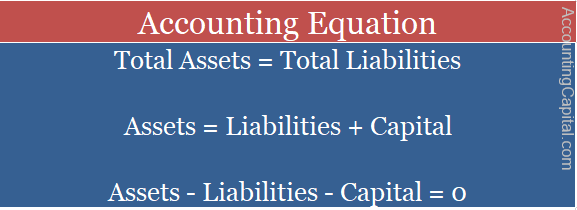# What is the Accounting Equation?

## Accounting Equation

It wouldn’t be wrong to say that this equation is the basis of all accounting. The Accounting Equation is based on the dual aspect concept of accounting, which says that every transaction has two aspects, debit and credit, and for every debit, there is equal and opposite credit.

This equation is also called the Balance Sheet Equation. It helps to prepare a balance sheet, which is the most vital step in creating financial statements.This relationship between Assets, Capital and Liabilities is called the Accounting Equation or the Balance Sheet Equation. In general, the expression Assets = Capital + Liabilities is termed as the Accounting Equation, but you can use any of the above relationships till the time you understand the fundamentals of the equation.

Therefore, at any point, the total number of assets of a firm is equal to the total number of liabilities. That is because the equation indicates that sources of funds are equal to the uses of funds. In other words, the equation means that capital and liabilities together are equal to assets at all times.

Key Highlights

• The accounting equation acts as a basis for accounting and uses the dual aspect principle of accounting.
• It is also known as the Balance Sheet Equation.
• According to the balance sheet equation, total assets are always equal to the sum of capital and external liabilities.

## Lite© – Learning Initiative Through Examples

A Business has assets worth 2,00,000 and owner’s equity of 69,000. What is the amount of liability that the business has?

Total assets = Total liabilities + Capital (or) Owner’s Equity

2,00,000 = Total liabilities + 69,000

2,00,000 – 69,000 = Total liabilities

1,31,000 = Total liabilities

Related Topic – What is a Journal (with template)?

## Application and Integrity of the Accounting Equation

In the below-mentioned example, we will deal with 2 different transactions that took place in a newly started business called Unreal Pvt Ltd. This example will help you clearly understand how a transaction affects the variables involved in an accounting equation and still maintains the integrity of the equation.

1. Unreal Pvt Ltd. starts a business with 1,000,000 cash (1 Million)

 Variables Affected Net Effect of Transaction Accounting Equation Capital & Assets Assets & Capital Both Increase Assets = Liabilities + Capital 1,000,000 (cash) = 0 + 1,000,000

Starting a business with 1 million means that the business owner introduced capital or in other words owner’s equity is 1M, which, in this case, was brought inside the business in the form of cash. Therefore, their cash increased by 1M and capital also increased simultaneously by the same amount.

2. They purchase raw material for 50,000 cash

 Variables Affected Net Effect of Transaction Accounting Equation Only Assets Raw material (Asset) increases and Cash (Asset) decreases  by 50k Assets = Liabilities + Capital 1M + 50k – 50k (Cash) = 0 + 1M

Unreal Pvt Ltd began operations by purchasing raw material for their business for 50,000 in cash. This transaction ultimately reduced 50k worth of cash and added 50k worth of raw material to the business.

Similarly, when other more complex transactions take place in business, the accounting equation will get affected each time, but it would still maintain its basic fundamental of assets being equal to the sum of capital and liabilities.